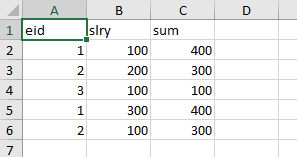# Sum the total of salary when emp id are same and without removing duplicate

Hello All,
I have a condition in excel/datatable,
I have duplicate ids but data for these rows is different, I need to check if id are same, if they are same then sum up the totals of duplicate id like here id 1 and 2 are duplicate, so sum of id 1 + sum of second id 1 is 100+300=400 and 400 should be updated in both rows.

example
E_id slry sum
1 100 100
2 200 200
3 100 100
1 300 300
2 100 100

I want it as

eid Slry sum
1 100 400
2 200 300
3 100 100
1 300 400
2 100 300

can some one suggest any way of doing it?

Could you please elaborate your requirement so that solution can be found.
Regards,

In the left hand side of assign acitivity you should use dt2, in the right hand side you should use Linq.
Also see below updated linq:
(From row in dt1.AsEnumerable
Group row by sc = row(“E_id”).ToString()
Into grp = Group
Let total= grp.sum(Function (x) Convert.ToDouble(x(“sum”).ToString()))
Let result = New-Object() {grp(0)(“E_id”),total}

1 Like

Hi,

Hope the following sample helps you.

``````dt = dt.AsEnumerable.Select(Function(r) dt.Clone.LoadDataRow({r(0),r(1),dt.AsEnumerable.Where(Function(r2) r2("eid").ToString=r("eid").ToString).Sum(Function(r2) CInt(r2("Slry").ToString))},False)).CopyToDataTable
``````

Sample20230530-7L.zip (8.3 KB)

Regards,

@Yoichi Actually, it also removing the duplicate rows, In my requiremnt, I dont want to remove the duplicate row, instead of removing, I want to update sum of all totals of same id in every duplicate id

Hi,

Did you run the above sample? It returns the following sheet as result.Regards,

@Yoichi It worked, you are best

1 Like

This topic was automatically closed 3 days after the last reply. New replies are no longer allowed.